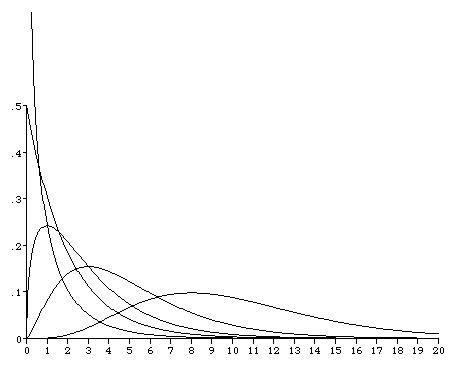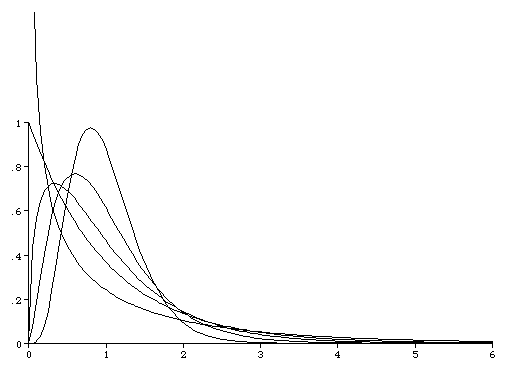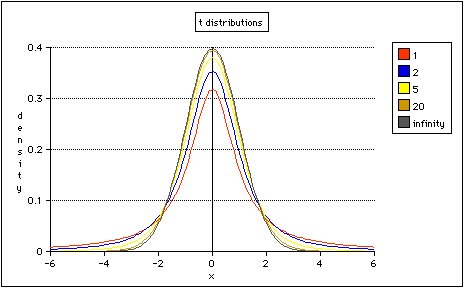# The Chi-square and t Distributions

## Chi-square Distribution

The graph below shows the Chi-square probability density function plotted for 1, 2, 3, 5 and 10 degrees of freedom. Compare this plot with Figure 6.8 on page 170 of Rosner, 4th Edition, which is not drawn as accurately, and Figure 6.8 on page 188 of Rosner, 5th Edition.This is the same as the plot above, but to make the shapes easier to compare, I have re-scaled each distribution to have unit mean.## Student's t Distribution

The graph below shows the Student's t probability density function plotted for 1, 2, 5, 20 and infinite degrees of freedom; t on infinite degrees of freedom is the same as the standard normal distribution.

This graph is accurately drawn. Compare it with Figure 6.7 on page 166 of Rosner, 4th Edition, which is very badly drawn, and Figure 6.6 on page 179 of Rosner, 5th Edition.